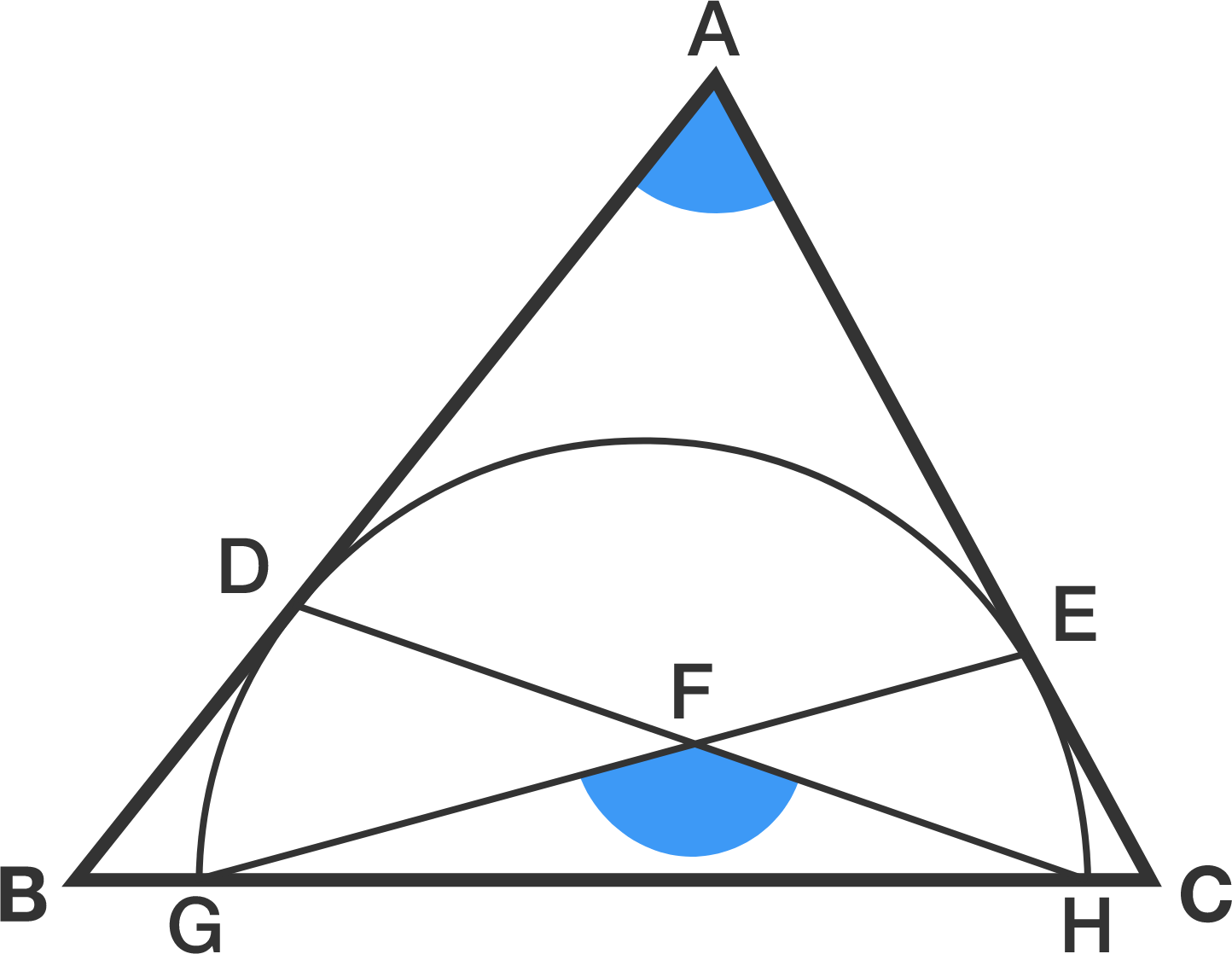# Inscribed Semicircle Riddle

Geometry Level 2As shown in the diagram, triangle $ABC$ inscribes a semi-circle such that $AB$ and $AC$ are tangents at points $D$ and $E,$ respectively.

$GH$ is the diameter of the semi-circle, and point $F$ is the intersection of $DH$ and $EG.$

If the 2 angles highlighted in blue are equal, find that angle in degrees.


Note: The diagram is not drawn to scale.

×